Deepika Singh

# Create Conditional Calculations with Built-in Functions in Tableau

• Jun 3, 2020
• 26,412 Views
• Jun 3, 2020
• 26,412 Views
Data
Data Analytics
Tableau

## Introduction

Testing the logical scenarios of variables is a common task in data science and business intelligence. This allows us to determine if a certain condition is true or false (Boolean logic), thereby providing useful insights. In this guide, you will learn how to create conditional calculations with built-in functions in Tableau.

# Data

Conditional calculations can be applied to both dimensions and measures. This guide will be using the Sample Superstore data source available in the Tableau repository.

In the subsequent sections, you will implement several built-in functions including `IF`, `IFELSE`, `CASE`, and `ISDATE`.

## ISDATE

The `ISDATE` function is used to check whether the string value passed in the function is a date or not. This function is useful in scenarios where the data source has fields in string format. The syntax of the function is `ISDATE(String value)`, and the output is a Boolean expression—`TRUE` or `FALSE`.

The first step is to go to the Analysis tab and select the Create Calculated Field option, as shown below.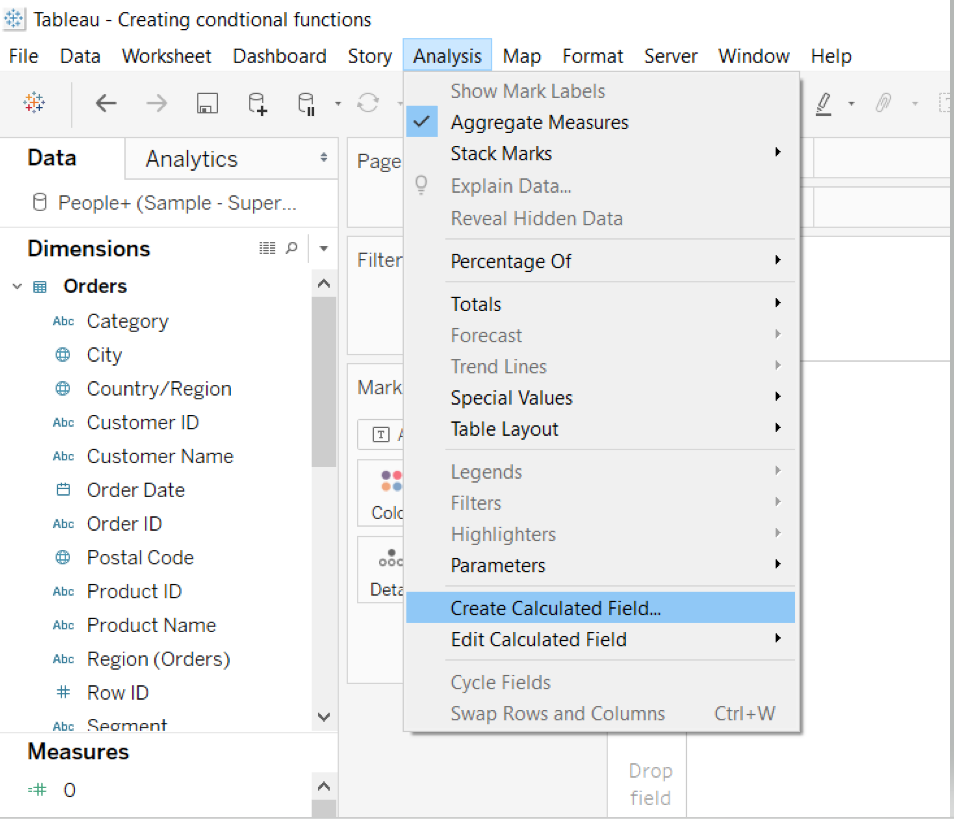The next step is to name the calculated field `ISDATE False E.g`. Next, write the expression as shown below, and click Ok. The calculation is valid because the datatype is string for the category.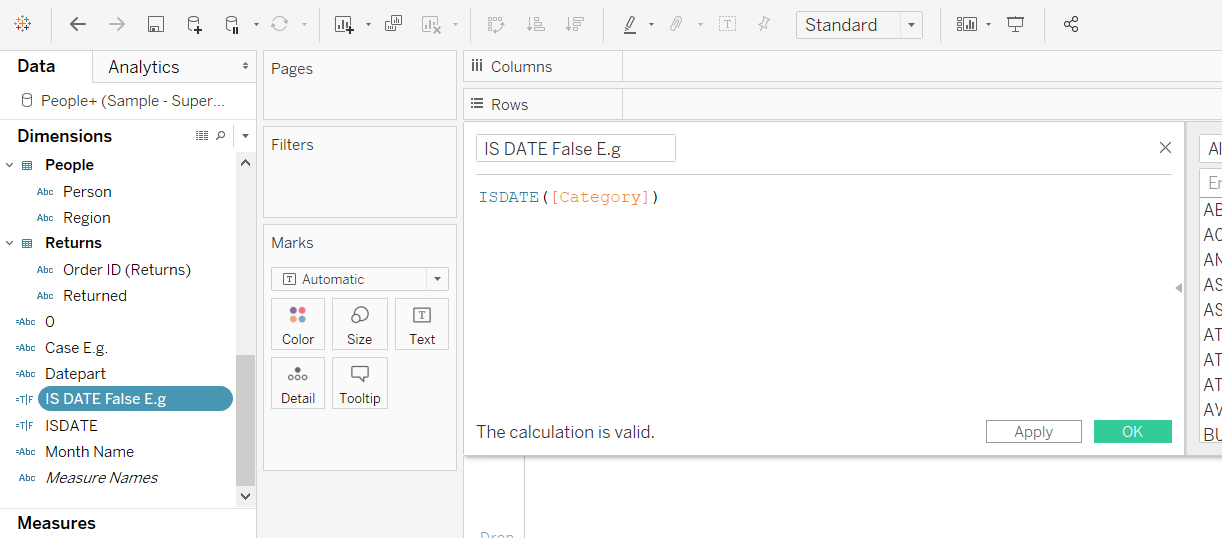Drag this calculated field into the view and check the output. The result will be False because `Category` is not a date type variable.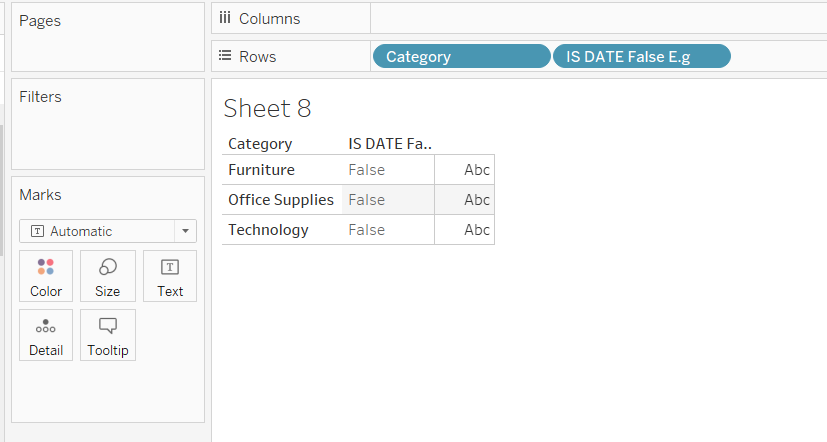If the variable was a date type, the result would have been True. To illustrate this, create another calculated field as above and name it `ISDATE True E.g.`. Next, write the calculation as shown below.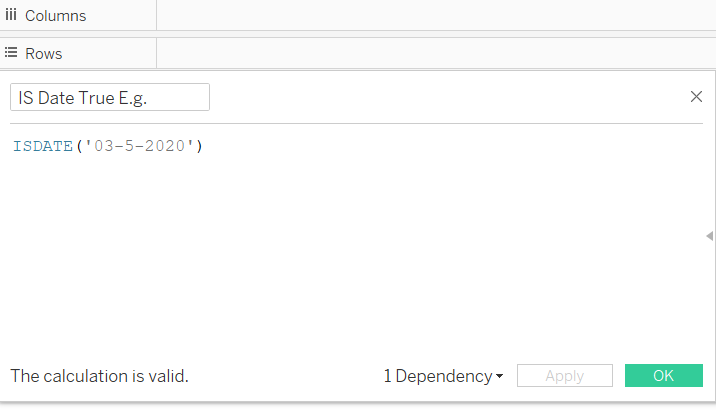Click on OK and drag the calculation into the view. The output will be True as the condition has been satisfied.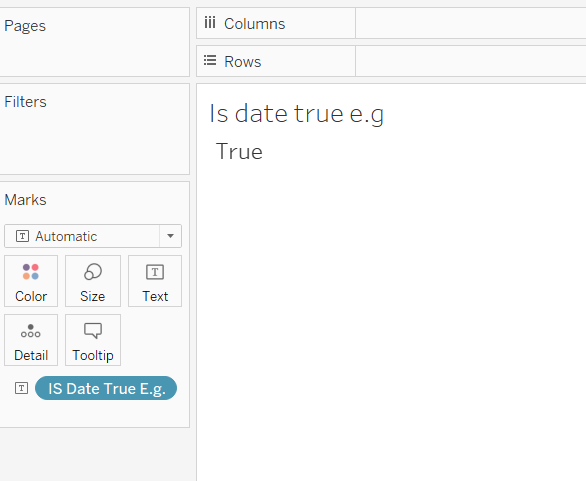## IIF

The `IIF` function is used to return the true output if the expression is met, otherwise it returns false or null as the output. The first step is to create a calculated field and name it `IIF example`. Enter the logic in the calculated field as shown below.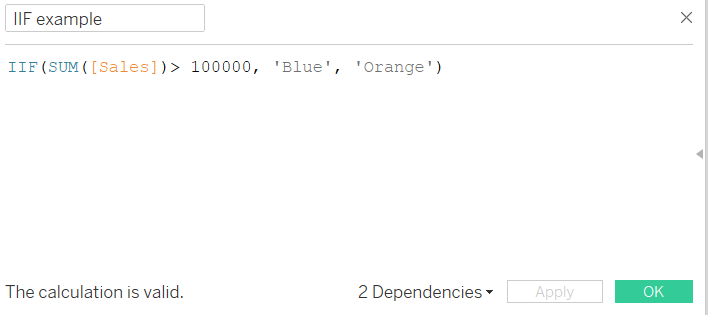Next, drag `Sub-Category` into the Columns shelf and `Sales` into the Rows shelf. Turn on the label to display the values.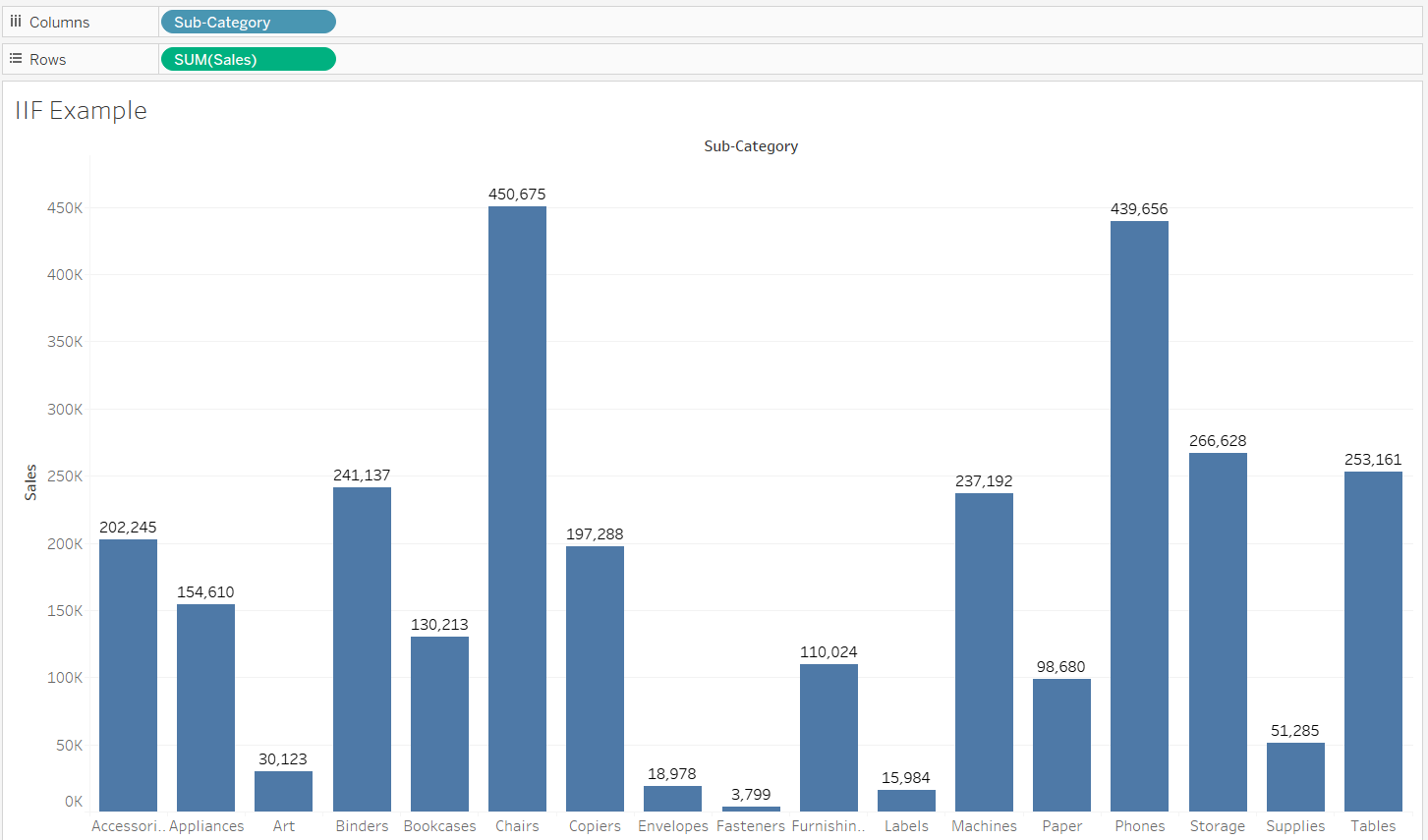Next, drag the calculated field `IIF Example` into the Color option of the Marks card.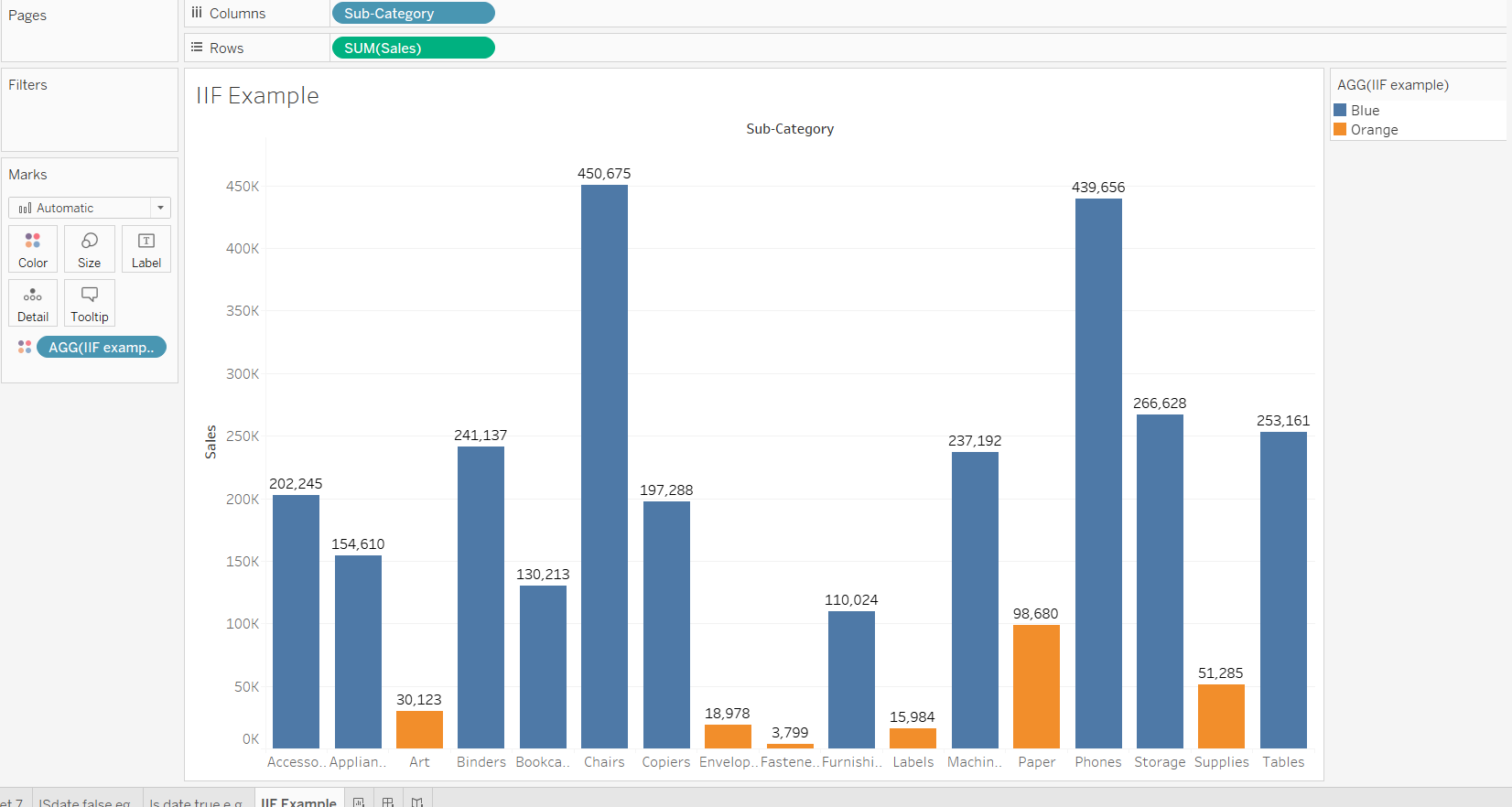The output above shows that the bar chart is demonstrating the color as specified in the logic.

## IF THEN

The `IF` function allows the user to construct only one condition, and if the condition is satisfied, the function returns the output. The extended argument `THEN` is used to return the result, given the expression in the `IF` part is satisfied.

To start, create a calculated field named `IF example`, and write the logical expression as shown below. This expression will return the `Sales` amount only if the `Category` condition is satisfied.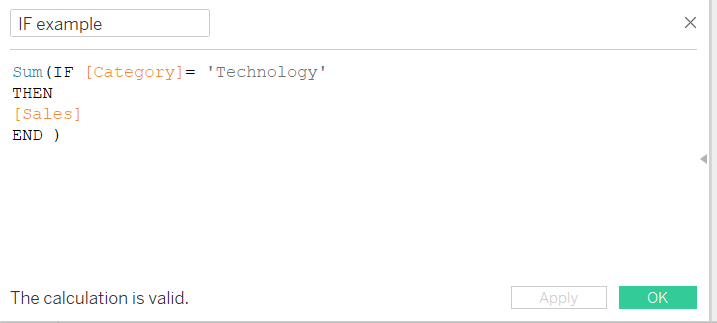Drag the `Category` into the Rows shelf and the calculated field, `IF example`, into the Text option of the Marks shelf.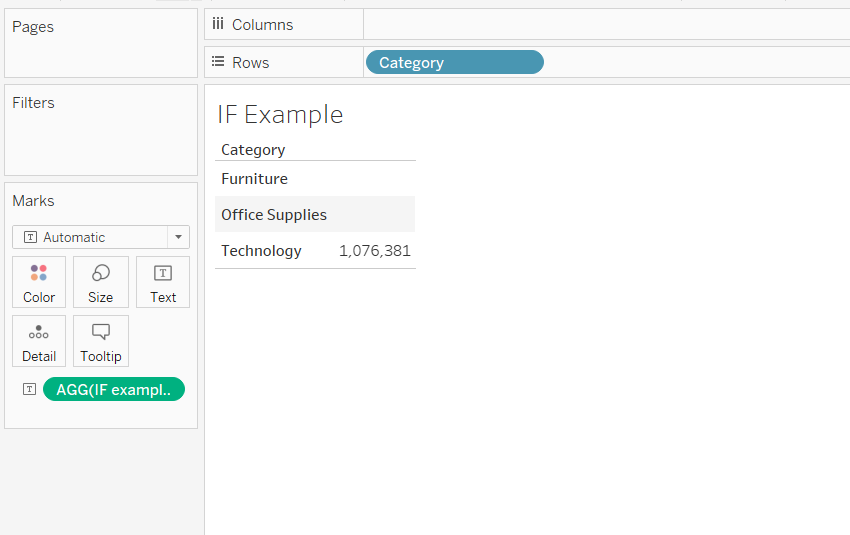The output above shows that the other two categories are blank, and sales is displayed only for the `Technology` category.

## IF ELSE

This is an upgraded version of the previous `IF THEN` function. You can create two conditions using this function. To start, create a calculated field named `IF ELSE eg`, and write the logical expression as shown below. This expression returns the sales value when the condition is met, otherwise it returns a value of zero.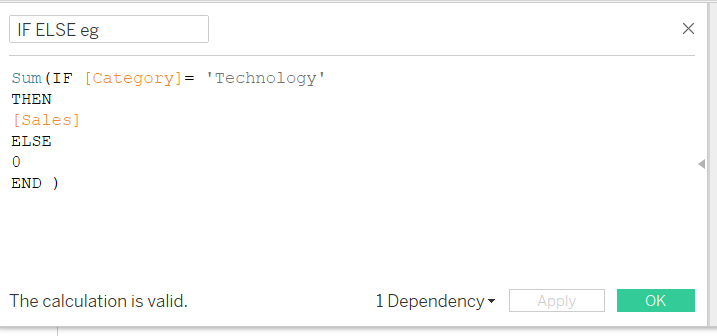Next, drag the `Category` into the Rows shelf and the calculated field into the Text option of the Marks shelf.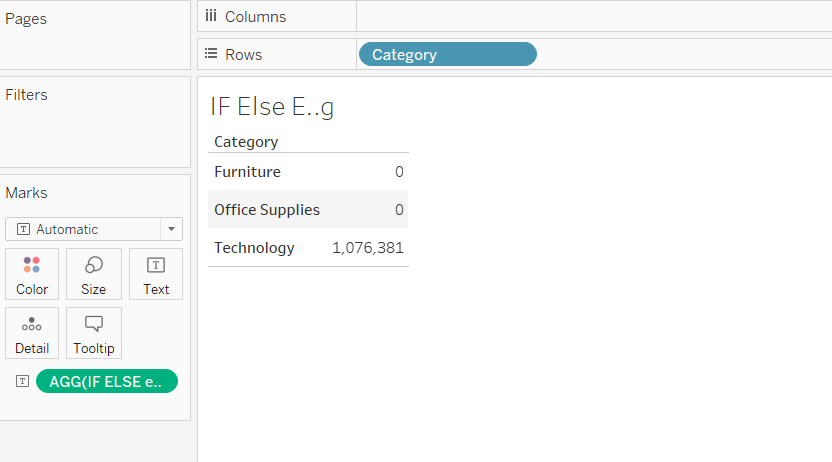The output above shows that the sales value is displayed only for the `Technology` category.

## CASE

The `CASE` function evaluates the expression mentioned in the conditions, scans through the values, and returns the value that matches the expression. To start, create a calculated field named `Case E.g.`, and write the logical expression as shown below.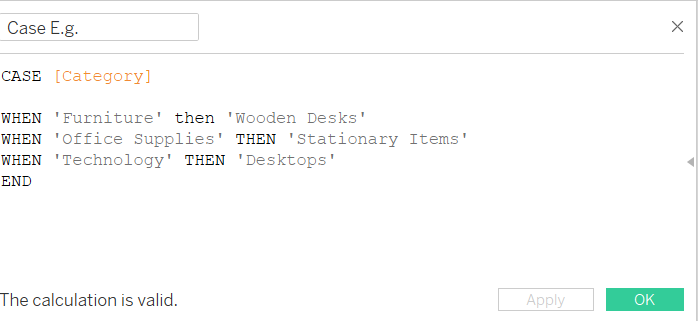The next step is to drag the `Category` and the calculated field, `Case E.g.`, into the Rows shelf. Also, place the `Case E.g.` variable into the Text option of the Marks shelf. This will generate the required output.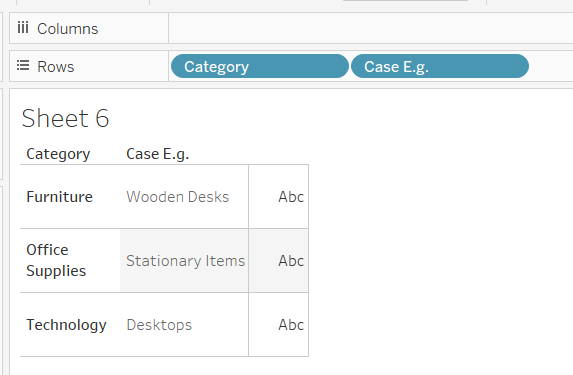## Conclusion

In this guide, you learned how to create conditional calculations using built-in functions in Tableau. You learned several functions which are commonly used while working with data. This will help strengthen your descriptive analytics capabilities.